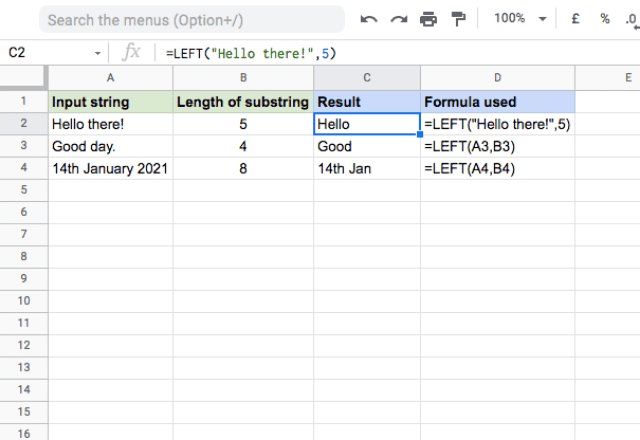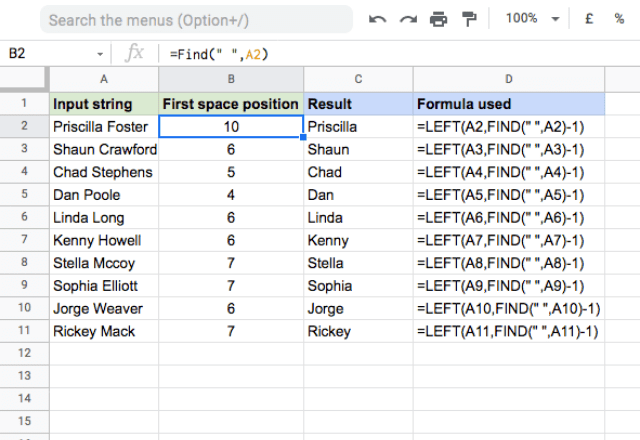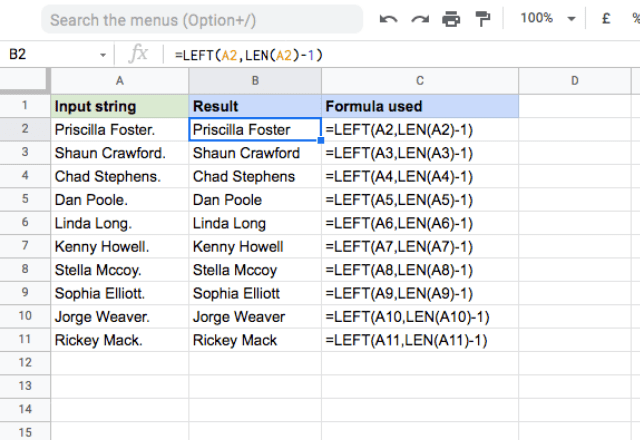# How to use the LEFT formula in Google SheetsSpreadsheet applications today are extremely versatile and useful tools. While they are known primarily for handling numbers, they also provide many ways to operate with text values. In this post, you’ll learn about the LEFT function in Google Sheets. This function helps extract a substring from the beginning of a specified string of text.

=LEFT(string, [number_of_characters])
• string – is the input text from which the formula returns the left portion.
• number_of_characters – [ OPTIONAL – 1 by default ] – this is the number of characters that the formula should return from the left side of the string.

### Usage: LEFT function in Google Sheets

A quick look at the syntax will reveal that you just need an input string and the length of the substring to extract. That sounds relatively simple, but I’ll give a few examples to help reinforce the concept. Please take a look at the image below.In each of these examples, the function begins at the start (left) of the text string and extracts the specified number of characters. The function itself is quite simple to use; the last example, for instance, uses the formula =LEFT(A4,B4).

Like most other Google Sheets functions, the LEFT function can accept both direct values and references to cells that hold these values. I’ve illustrated this in the examples, by typing the string and number_of_characters directly into the function in the first example, and then using cell references for the others.

Regardless of the approach to inputting the formulas, you’ll notice that the function always extracts the substring from the left side. In the first example, I have the string “Hello there!”. The substring that is 5 characters long from the left is “Hello”, which the formula has returned. The same logic applies to the other examples.

#### Using LEFT with FIND

So far I have used static numbers for the number_of_characters parameter. It certainly gets more interesting if you make this dynamic. Suppose that you have to extract the first names from a list of full names. Given that each first name varies in length, it is not possible to use a constant value for number_of_characters. All you know is that the first and last names are separated by a space character.

In this situation, you can make use of the FIND formula. It returns the position at which a string is first found within a text. Consider the case of Shaun Crawford. If you count manually, the space character is in the sixth position. That is exactly what the FIND formula returns. Since you know the space is the sixth character, you also know that to extract the first name “Shaun”, the function needs to take the first five characters of the string. By plugging in the FIND function and subtracting 1 (so that the space isn’t included) you can extract the first name.The formula used here is =LEFT(A2,FIND(” “,A2)-1). This approach works for all the names regardless of their length, since the FIND function creates a dynamic input to the number_of_characters parameter.

The FIND function allows you to use this approach whenever your data is in a consistent format that allows you to “find” a particular string each time. This could work for data that’s separated by a comma, a dash, a common phrase, or any other character or text string!

#### Using LEFT with LEN

You can also use the LEFT function to get rid of unwanted characters at the end of text strings. In this example, I again have a list of names, but they all have a period at the end. If you want to get rid of the period (and don’t want to manually delete each one) you can use the LEFT function in combination with the LEN function.

The LEN function returns the length of a text string; you can use it to generate the number_of_characters parameter within the LEFT function. By typing =LEFT(A2,LEN(A2)-1) you can specify the number_of_characters parameter to be “the length of the string, minus one character”. This way, no matter what the length of the string is, you’ll always be removing the last character. You can see that this works well – the periods have been removed!You can use this approach in a variety of situations. And if you want to remove more that one character from the end of the string, just change the -1 in the formula to whatever number you want!

Editor’s note: This is a revised version of a previous post that has been updated for accuracy and comprehensiveness.